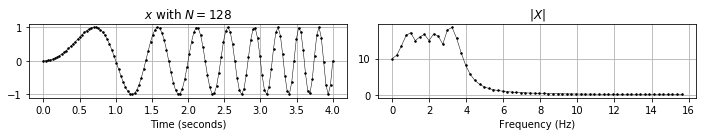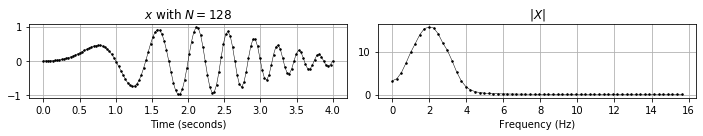# Exercise: Fourier Analysis of Signals¶

## Prerequisites¶

To work on this exercise, you should first work through the Lecture on Fourier Analysis of Signals. In particular, go through the notebooks mentioned in the lecture overview.

## Tasks¶

### Task: Complex Numbers¶

Go through the PCP notebook on Complex Numbers and complete exercises 1 and 2.

### Task: Exponential Function¶

Go through the PCP notebook on the Exponential Function and complete exercise 1 and optionally exercise 3.

### Task: Sampling, Aliasing, Beating¶

Go through the PCP notebook on Signals and Sampling and complete exercises 1 and 2. Be sure that you understand the concepts of sampling, aliasing and beating.

Take some audio file (e.g. your favorite song) and listen to downsampled versions of it at different sampling rates. Can you find signals for which the effect is more pronounced? And can you find signals for which the original and the downsampled version sound identical?

### Task: Quantization¶

Go through the FMP notebook on Quantization.

Again, take some audio file and experiment with different quantization levels. At which quantization strength do you begin to hear quantization noise?

### Task: DFT and FFT¶

Go through the PCP notebook on the DFT and FFT and complete exercises 1, 2, 3 and optionally exercise 4 for the DFT. You may check the FMP notebook on DFT and FFT for more explanations of the concepts.

Explain in your own words: What happens to a signal $x$ when it is multiplied with the matrix $\mathrm{DFT}_N$? Check your understanding by looking at the visualizations of $\mathrm{Re}(\mathrm{DFT}_N)$ and $\mathrm{Im}(\mathrm{DFT}_N)$ and answering the following questions:

• Why do both matrices show a 2-by-2 pattern?
• Why are the quadrants of the 2-by-2 pattern in $\mathrm{Im}(\mathrm{DFT}_N)$ separated by white lines?
• In $\mathrm{Re}(\mathrm{DFT}_N)$, you can see circles which are, alternatingly, blue-red-blue-red... Why do they appear?
• In $\mathrm{Im}(\mathrm{DFT}_N)$, you can see colored circles, too. Observe, however, that the colors do not match between neighboring quadrants. Why does this happen?

### Optional Task: Frequency Grid¶

Go through the FMP notebooks on Frequency Grid Density up until, but not including, "Frequency Interpolation for STFT" and Frequency Grid Interpolation up until, but not including, "STFT with Increased Frequency Grid Resolution".

Answer the following questions:

• What do the following terms refer to? Do they refer to the same thing or how are they different?
• Frequency Grid Density
• Frequency Resolution
• DFT approximation quality
• Consider the two techniques introduced in the two notebooks. How do they affect frequency grid density, frequency resolution and DFT approximation quality?
• When would you prefer one technique over the other?

### Task: FFT¶

Read the FMP notebook on DFT and FFT and try to understand the FFT algorithm in depth. For this, also read Section 2.4.3 of the text book. Be able to answer the following questions

• Why do we have $\sigma_M = \sigma_N^2$?
• Why do we say that the FFT algorithm is a recursive algorithm?
• Why does naive application of the DFT take operations in the order of $N^2$?
• How is this complexity reduced in the FFT algorithm?
• Optional Task: Prove that FFT and naive application of the DFT yield the same result.

## Question & Answer Session¶

### Why does the magnitude spectrum of the chirp signal have show oscillations?¶

These oscillations can be seen as ripple artifacts that arise because sinusoids at many frequencies interfere to create the chirp and the zero-extension of the signal outside of the finite chirp. The artifacts can be reduced by applying a different window function, such as a Gaussian window as seen below.

In :
import scipy.signal
import numpy as np
import matplotlib.pyplot as plt

def fft(x):
"""Compute the fast Fourier transform (FFT)

Notebook: PCP_dft.ipynb

Args:
x: Signal to be transformed

Returns:
X: Fourier transform of x
"""
x = x.astype(np.complex128)
N = len(x)
log2N = np.log2(N)
assert log2N == int(log2N), 'N must be a power of two!'
X = np.zeros(N, dtype=np.complex128)

if N == 1:
return x
else:
this_range = np.arange(N)
A = fft(x[this_range % 2 == 0])
B = fft(x[this_range % 2 == 1])
range_twiddle_k = np.arange(N // 2)
sigma = np.exp(-2j * np.pi * range_twiddle_k / N)
C = sigma * B
X[:N//2] = A + C
X[N//2:] = A - C
return X

def plot_signal_dft(t, x, X, ax_sec=False, ax_Hz=False, freq_half=False, figsize=(10, 2)):
"""Plotting function for signals and its magnitude DFT

Notebook: PCP_dft.ipynb

Args:
t: Time axis (given in seconds)
x: Signal
X: DFT
ax_sec: Plots time axis in seconds
ax_Hz: Plots frequency axis in Hertz
freq_half: Plots only low half of frequency coefficients
figsize: Size of figure
"""
N = len(x)
if freq_half is True:
K = N // 2
X = X[:K]
else:
K = N

plt.figure(figsize=figsize)
ax = plt.subplot(1, 2, 1)
ax.set_title('$x$ with $N=%d$' % N)
if ax_sec is True:
ax.plot(t, x, 'k', marker='.', markersize='3', linewidth=0.5)
ax.set_xlabel('Time (seconds)')
else:
ax.plot(x, 'k', marker='.', markersize='3', linewidth=0.5)
ax.set_xlabel('Time (samples)')
ax.grid()

ax = plt.subplot(1, 2, 2)
ax.set_title('$|X|$')
if ax_Hz is True:
Fs = 1 / (t - t)
ax_freq = Fs * np.arange(K) / N
ax.plot(ax_freq, np.abs(X), 'k', marker='.', markersize='3', linewidth=0.5)
ax.set_xlabel('Frequency (Hz)')

else:
ax.plot(np.abs(X), 'k', marker='.', markersize='3', linewidth=0.5)
ax.set_xlabel('Frequency (index)')
ax.grid()
plt.tight_layout()
plt.show()

def generate_chirp_linear(t0=0, t1=1, N=128):
"""Generation chirp with linear frequency increase

Notebook: PCP_DFT.ipynb

Args:
t_0: Start time (seconds)
t_1: End time (seconds)
N: Number of samples

Returns:
x: Generated chirp signal
t: Time axis (in seconds)
"""
t = np.linspace(t0, t1, N)
x = np.sin(np.pi * t ** 2)
return x, t

def generate_chirp_plot_signal_dft(t0, t1, N, figsize=(10, 2), apply_gaussian=False):
x, t = generate_chirp_linear(t0=t0, t1=t1, N=N)
if apply_gaussian:
w = scipy.signal.windows.gaussian(N, std=N/4)
x *= w
X = fft(x)
plot_signal_dft(t, x, X, ax_sec=True, ax_Hz=True, freq_half=True)

generate_chirp_plot_signal_dft(t0=0, t1=4, N=128)
generate_chirp_plot_signal_dft(t0=0, t1=4, N=128, apply_gaussian=True)